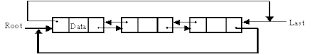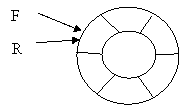## Thursday, December 6, 2007

### Circular Queue Data Structure

In a standard queue data structure re-buffering problem occurs for each dequeue operation. To solve this problem by joining the front and rear ends of a queue to make the queue as a circular queue
Circular queue is a linear data structure. It follows FIFO principle.
• In circular queue the last node is connected back to the first node to make a circle.
• Circular linked list fallow the First In First Out principle
• Elements are added at the rear end and the elements are deleted at front end of the queue
• Both the front and the rear pointers points to the beginning of the array.
• It is also called as “Ring buffer”.
• Items can inserted and deleted from a queue in O(1) time.

Circular Queue can be created in three ways they are
· Using arrays
It is an extension for the basic single linked list. In circular linked list Instead of storing a Null value in the last node of a single linked list, store the address of the 1st node (root) forms a circular linked list. Using circular linked list it is possible to directly traverse to the first node after reaching the last node.
The following figure shows circular single linked list:In double linked list the right side pointer points to the next node address or the address of first node and left side pointer points to the previous node address or the address of last node of a list. Hence the above list is known as circular double linked list.
The following figure shows Circular Double linked list :-Algorithm for creating circular linked list :-
Step 1) start
Step 2) create anode with the following fields to store information and the address of the next node.
Structure node
begin
int info
pointer to structure node called next
end
Step 3) create a class called clist with the member variables of pointer to structure nodes called root, prev, next and the member functions create ( ) to create the circular linked list and display ( ) to display the circular linked list.
Step 4) create an object called ‘C’ of clist type
Step 5) call C. create ( ) member function
Step 6) call C. display ( ) member function
Step 7) stop
Algorithm for create ( ) function:-
Step 1) allocate the memory for newnode
newnode = new (node )
Step 2) newnode->next=newnode. // circular
Step 3) Repeat the steps from 4 to 5 until choice = ‘n’
Step 4) if (root=NULL)
root = prev=newnode // prev is a running pointer which points last node of a list
else
newnode->next = root
prev->next = newnode
prev = newnode
Step 6) return
Algorithm for display ( ) function :-
Step 1) start
Step 2) declare a variable of pointer to structure node called temp, assign root to temp
temp = root
Step 3) display temp->info
Step 4) temp = temp->next
Step 5) repeat the steps 6 until temp = root
Step 6) display temp info
Step 7) temp=temp->next
Step 8) return
Using array
In arrays the range of a subscript is 0 to n-1 where n is the maximum size. To make the array as a circular array by making the subscript 0 as the next address of the subscript n-1 by using the formula subscript = (subscript +1) % maximum size. In circular queue the front and rear pointer are updated by using the above formula.
The following figure shows circular array:Algorithm for Enqueue operation using array
Step 1. start
Step 2. if (front == (rear+1)%max)
Print error “circular queue overflow “
Step 3. else
{ rear = (rear+1)%max
Q[rear] = element;
If (front == -1 ) f = 0;
}
Step 4. stop

Algorithm for Dequeue operation using array

Step 1. start
Step 2. if ((front == rear) && (rear == -1))
Print error “circular queue underflow “
Step 3. else
{ element = Q[front]
If (front == rear) front=rear = -1
Else
Front = (front + 1) % max
}
Step 4. stop

Hi, Thanks a lot, This post helped me to do my assignment. Its very simple and clear. Thanks

Unknown said...
This comment has been removed by the author.
Unknown said...

What is the drawback of circular queue

Unknown said...

Hi,thanks for this post.it helped me.

Unknown said...

This is very useful for me

^ Scroll to Top